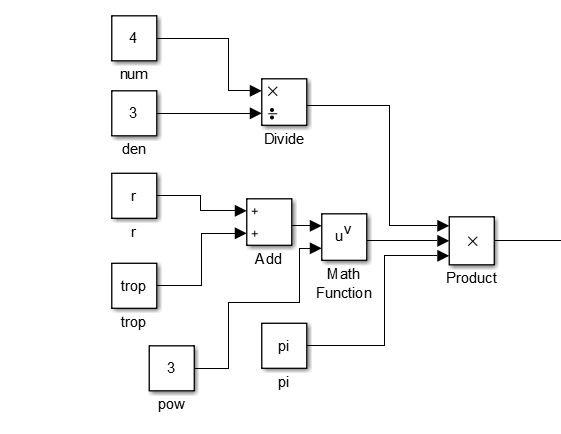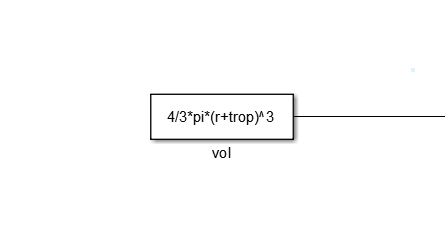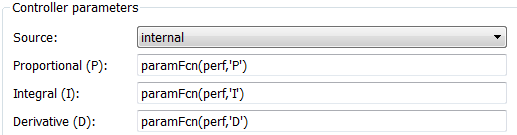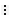Main Content

## Set Block Parameter Values

Blocks have numeric parameters that determine how they calculate output values. To control the calculations that blocks perform, you can specify parameter values. For example, a Gain block has a Gain parameter, and a Transfer Fcn block has multiple parameters that represent the transfer function coefficients.

You can use numbers, variables, and expressions to set block parameter values. Choose a technique based on your modeling goals. For example, you can:

• Share parameter values between blocks and models by creating variables.

• Control parameter characteristics such as data type and dimensions by creating parameter objects.

• Model an algorithm as code by using mathematical expressions.

Set block parameters using the Parameters tab in the Model Data Editor (on the Modeling tab, click Model Data Editor), the Property Inspector (on the Modeling tab, under Design, click Property Inspector), or the block dialog box. For more information, see Add Blocks and Set Parameters. To set block sample times, see Specify Sample Time.

Tip

You can use the Model Explorer to make batch changes to many block parameter values at once. For more information, see Model Explorer.

### Programmatically Access Parameter Values

To programmatically access block parameter values, use the `get_param` and `set_param` functions. You can use this technique to:

• Construct a model programmatically.

• Adjust parameter values during a simulation run when you simulate a model programmatically.

To sweep parameter values between simulation runs by using a script, use `Simulink.SimulationInput` objects instead of `get_param` and `set_param`. See Optimize, Estimate, and Sweep Block Parameter Values.

Suppose you create a model named `myModel` that contains a Constant block named My Constant. Next, you use the block dialog box to set the Constant value parameter to `15`. To programmatically return the parameter value, use the function `get_param`. You specify the block path and the equivalent programmatic parameter name, `Value`.

`paramValue = get_param('myModel/My Constant','Value')`
```paramValue = 15```

To programmatically change the value, for example to `25`, use the function `set_param`. Use the character vector `'25'` as the input to the function.

`set_param('myModel/My Constant','Value','25')`

For a list of programmatic names of block parameters, see Block-Specific Parameters.

For more information about programmatic simulation, see Run Simulations Programmatically.

To avoid using the `get_param` and `set_param` functions, use the name of a MATLAB® variable or `Simulink.Parameter` object as the parameter value, and change the value of the variable or object at the command prompt. See Share and Reuse Block Parameter Values by Creating Variables.

### Specify Parameter Values

GoalBlock Parameter ValueDescription

Store the parameter value in the model file.

`2.3`

```[1.2 2.3 4.5; 7.9 8.7 6.5]```

`2 + 3i`

Literal numeric value. Specify a scalar, vector, matrix, or multidimensional array. Use `i` to specify complex values.

• Access the parameter value without having to locate or identify the block in the model.

• Change the parameter value without having to modify the model file.

• Share the parameter value between blocks or between models.

• Identify the parameter by a specific name when sweeping or tuning the value.

`myVar`

MATLAB variable that exists in a workspace.

For more information, see Share and Reuse Block Parameter Values by Creating Variables.

• Avoid name clashes between workspace variables.

• Organize parameter values using hierarchies and meaningful names.

• Reduce the number of workspace variables that a model uses.

`myParam.a.SpeedVect`

Field of parameter structure.

For more information, see Organize Related Block Parameter Definitions in Structures.

Use a portion of a matrix or array variable. For example, set the parameters of a n-D Lookup Table block.

`myMatrixParam(:,2)`

Index operation.

• Define parameter characteristics, such as data type, complexity, units, allowed value range, and dimensions, separately from the parameter value.

• Define a system constant with custom documentation.

• Create a tunable parameter in the generated code.

• Set the value of a variable to a mathematical expression involving constants and other variables.

`myParam`

Parameter object.

For more information, see Use Parameter Objects.

• Express a parameter value as a mathematical relationship between known physical constants instead of as an unidentifiable literal number.

• Reduce block population in a model.

• Model an obscure or trivial calculation by using code instead of blocks.

• Use MATLAB operators and functions to perform calculations.

• Write a custom MATLAB function that calculates parameter values.

`5^3.2 - 1/3`

```myParam * myOtherparam + sin(0.78*pi)```

`myFun(15.23)`

Expression or custom function.

For more information, see Use Mathematical Expressions, MATLAB Functions, and Custom Functions.

Specify a block parameter value by using a data type other than `double`.

`15.23`

`single(15.23)`

`myParam`

Typed or untyped expression, numeric MATLAB variable, or parameter object.

For more information about controlling parameter data types, see Control Block Parameter Data Types.

#### Use Parameter Objects

Parameter objects are `Simulink.Parameter` objects and objects of the subclasses that you create. The parameter object exists in a workspace such as the base workspace or a data dictionary.

You can use parameter objects to define system constants. For example, use a parameter object to represent the radius of the Earth. Use the properties of the object to specify the physical units and to document the purpose of the value.

Create parameter objects to prepare your model for code generation. You can configure parameter objects to appear as tunable global variables in the generated code. You can also control the parameter data type through the object.

To create and use parameter objects in models, see Data Objects. For information about using variables to set block parameter values, see Share and Reuse Block Parameter Values by Creating Variables.

#### Use Mathematical Expressions, MATLAB Functions, and Custom Functions

You can set a block parameter value to an expression that calls MATLAB functions and operators such as `sin` and `max`. You can also call your own custom functions that you write on the MATLAB path.

Suppose that a section of your block algorithm uses variables to calculate a single constant number used by the rest of the algorithm. You can perform the calculation by creating multiple blocks.Instead, create a single Constant block that uses an expression written in MATLAB code. This technique reduces the size of the block algorithm and improves readability.You can model a complicated portion of an algorithm by using an expression instead of many blocks. To operate on an existing signal, use a mathematical expression as the value of a parameter in an algorithmic block, such as the Gain parameter of a Gain block.

With expressions, you can also call your custom functions to set block parameter values. Suppose that you write a MATLAB function that calculates optimal P, I, and D parameters for a control algorithm by accepting a single input number.You can parameterize a PID Controller block by using the function to set the parameter values.To make the best use of expressions, consider these tips:

• If you use variables and parameter objects, you can explicitly model the algebraic relationships between the real-world quantities that the variables and objects represent. Use expressions in parameter objects as described in Set Variable Value by Using a Mathematical Expression.

• While you edit an expression in a block parameter value, to navigate to the documentation for a function, use the buttonnext to the parameter value. You can also navigate to the source code of a custom function.

### Considerations for Other Modeling Goals

Choose a technique to set block parameter values based on your modeling goals.

GoalFeatures or ProductsBest Practice
Run multiple simulations quickly.`Simulink.SimulationInput` objects and the `sim` functionUse variables or parameter objects to set block parameter values. This technique helps you to assign meaningful names to the parameters and to avoid having to identify or locate the blocks in the model. See Optimize, Estimate, and Sweep Block Parameter Values.
Sweep parameter values during testing.Simulink® Test™Use variables or parameter objects to set block parameter values. Use iterations and parameter overrides to run multiple tests. See Parameter Overrides (Simulink Test) and Test Iterations (Simulink Test).
Estimate and optimize parameter values.Simulink Design Optimization™

Use variables or parameter objects to set block parameter values.

To estimate or optimize a parameter that uses a data type other than `double`, use a parameter object to separate the value from the data type.

For parameter estimation, see Parameter Estimation (Simulink Design Optimization). For response optimization, see Optimize Model Response (Simulink Design Optimization).

Generate code from a model. Simulate an external program through SIL/PIL or External mode simulations.Simulink Coder™

Use parameter objects to set block parameter values. This technique helps you to declare and identify tunable parameters in the generated code and to control parameter data types. See Create Tunable Calibration Parameter in the Generated Code (Simulink Coder).

When you use expressions to set block parameter values, avoid using operators and functions that result in loss of tunability in the generated code. See Tunable Expression Limitations (Simulink Coder).

## Support

#### Model-Based Design for Embedded Control Systems

Download white paper